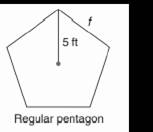Chapter 11.CR, Problem 4CRElementary Geometry For College St...

7th Edition
Alexander + 2 others
ISBN: 9781337614085

Solutions

Chapter
SectionElementary Geometry For College St...

7th Edition
Alexander + 2 others
ISBN: 9781337614085
Textbook Problem

In Review Exercises 1 to 4, state the ratio needed, and use it to find the measure of the indicated line segment to the nearest tenth of a unit.To determine

To find:

The measure of the indicated line segment to the nearest tenth of a unit.

Explanation

Procedure used:

In any right triangle ABC with mA=θ we have the following ratios.

sinθ=OppositeHypotenuse

Calculation:

Given:

The above figure is a pentagon with radius r=5 ft.

Let us name the given figure of pentagon as ABCDE.

Now,

OC=r=5 ft, and

CD= ft.

Consider the radius of the pentagon OD, adjacent to the radius OC.

Calculate the angle subtended between the radii.

Now, the angle between the radii OC and OD is given by

=360°5

=72°

Thus,

mCOD=72°

Still sussing out bartleby?

Check out a sample textbook solution.

See a sample solution

The Solution to Your Study Problems

Bartleby provides explanations to thousands of textbook problems written by our experts, many with advanced degrees!

Get Started

let f(x) = x3 + 5, g(x) = x2 2, and h(x)= 2x + 4. Find the rule for each function. 3. fg

Applied Calculus for the Managerial, Life, and Social Sciences: A Brief Approach

Evaluate the integral. 01coshtdt

Single Variable Calculus: Early Transcendentals, Volume I

Find the mean for the scores in the following frequency distribution table: X f 6 1 5 4 4 2 3 2 2 1

Essentials of Statistics for The Behavioral Sciences (MindTap Course List)

In Problems 1-20, evaluate the improper integrals that converge.

Mathematical Applications for the Management, Life, and Social Sciences

limx2x2(x1)1x = _____. a) 4 b) 1 c) 0 d) does not exist

Study Guide for Stewart's Single Variable Calculus: Early Transcendentals, 8th

Find the unit tangent vector for at t = –1.

Study Guide for Stewart's Multivariable Calculus, 8th